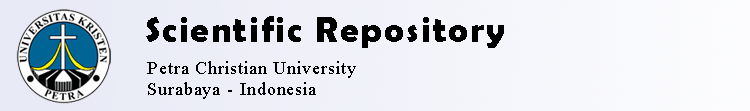# Learning Curve as a Knowledge-based Dynamic Fuzzy Set: A Markov Process Model

Halim, Siana and Intan, Rolly and Dewi, Lily Puspa (2018) Learning Curve as a Knowledge-based Dynamic Fuzzy Set: A Markov Process Model. In: IC4S, 21-10-2018 - 21-10-2018, Bangkok - Thailand.PDF Download (190Kb)
Official URL: http://ic4s.org

## Abstract

In the fuzzy set theory introduced by Zadeh (1965), membership de-gree of a fuzzy set is determined by a static membership function, i.e., it does not change over time. To improve this condition then Wang introduced the dy-namic fuzzy logic. In this concept, the membership degree of a fuzzy set is changing over the time. Intan and Mukaido (2002) introduced the knowledge-based fuzzy set, by means that the membership degree of a set is dependent on the knowledge of a person. Since the knowledge of a person is not static, the knowledge-based fuzzy set can be measured dynamically over time, so that we have the knowledge-based dynamic fuzzy set. In this paper, we approximate the learning process as a knowledge-based dynamic fuzzy set. We consider that the process of learning is dependent on the knowledge of a person from time to time so that we can model the learning process is a Markov process of dynamic knowledge. Additionally, using the triangular fuzzy number, we follow Yabuu-chi et al. (2014), for modelling the time difference in the dynamic knowledge fuzzy set as an autoregressive model of order one.

Item Type: Conference or Workshop Item (Paper) Learning curve, Fuzzy dynamic, Knowledge-based fuzzy set, Mar-kov process, Autoregressive Z Bibliography. Library Science. Information Resources > ZA Information resources Faculty of Industrial Technology > Industrial Engineering Department Admin 07 Feb 2019 17:16 11 Apr 2019 21:56 http://repository.petra.ac.id/id/eprint/18233

### Actions (login required)

 View Item